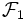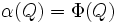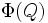# Morphism of fusion systems

Suppose$P_1, P_2$ are both finite$p$-groups (i.e., groups of prime power order for some prime number$p$). Suppose$\mathcal{F}_1$ is a fusion system on$P_1$ and$\mathcal{F}_2$ is a fusion system on$P_2$. A morphism of fusion systems from$\mathcal{F}_1$ to$\mathcal{F}_2$ is a pair$(\alpha,\Phi)$ where:
•$\alpha$ is a homomorphism of groups from$P_1$ to$P_2$.
•$\Phi$ is a covariant functor from the category$\mathcal{F}_1$ to the category$\mathcal{F}_2$.
• For every subgroup$Q \le P$,$\alpha(Q) = \Phi(Q)$. Here,$\alpha(Q)$ is the homomorphic image and$\Phi(Q)$ is the image of the object$Q \in \mathcal{F}_1$ under the covariant functor$\Phi$.
• If$\varphi:Q \to R$ is a morphism in$\mathcal{F}_1$, then$\Phi(\varphi) \circ \alpha = \alpha \circ \varphi$.